# GMAT Explanations

Official GMAT explanations tend to be clunky, confusing, afterthoughts of the GMAT universe and for the Official GMAT Practice Tests (1-6) there are no Official explanations. To help fill that gap we'll be updating this page with Official GMAT practice test explanations. If there are any GMAT puzzles for which you'd like a solution go ahead and add it to the comments section and we'll try to get to it faster. Happy studies!

From a group of 8 volunteers, including Andrew and Karen, 4 people are to be selected at random to organize a charity event. What is the probability that Andrew will be among the 4 volunteers selected and Karen will not?

(A) 3/7
(B) 5/12
(C) 27/70
(D) 2/7
(E) 9/35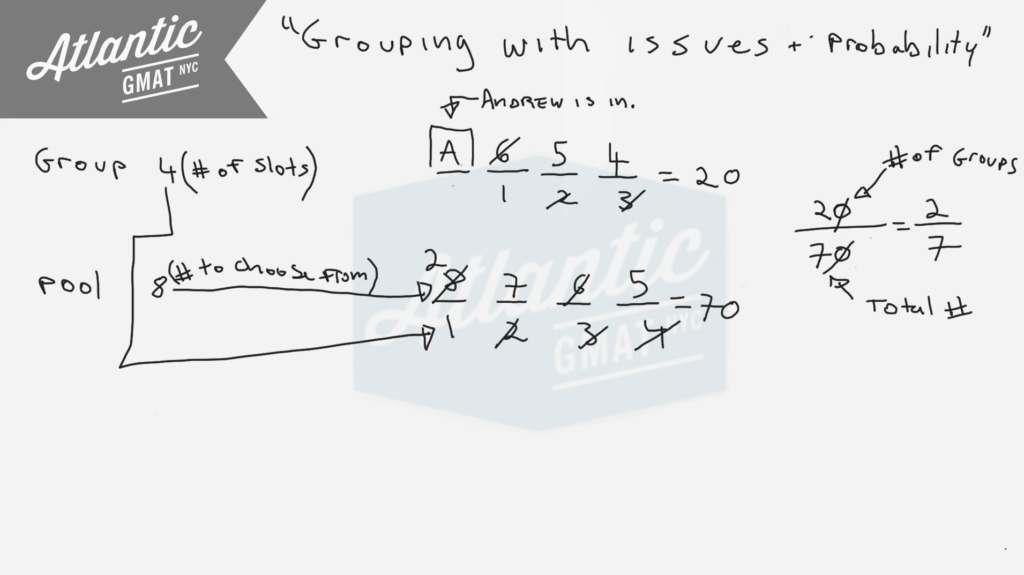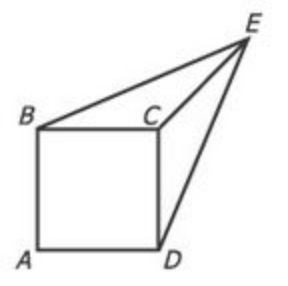In the figure above, each side of square ABCD has length 1, the length of line segment CE is 1, and the length of line segment BE is equal to the length of line segment DE. What is the area of the triangular region BCE?

(A) 1/3
(B) √2/4
(C) 1/2
(D) √2/2
(E) 3/4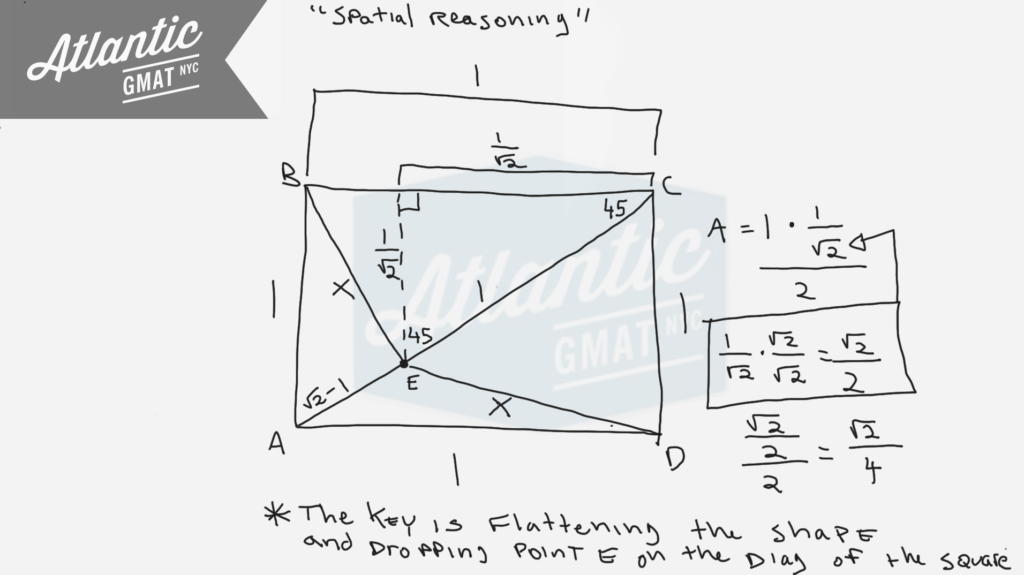A certain office supply store stocks 2 sizes of self-stick notepads, each in 4 colors: Blue, Green, Yellow or Pink. The store packs the notepads in packages that contain either 3 notepads of the same size and the same color or 3 notepads of the same size and of 3 different colors. If the order in which the colors are packed is not considered, how many different packages of the types described above are possible?

(A) 6
(B) 8
(C) 16
(D) 24
(E) 32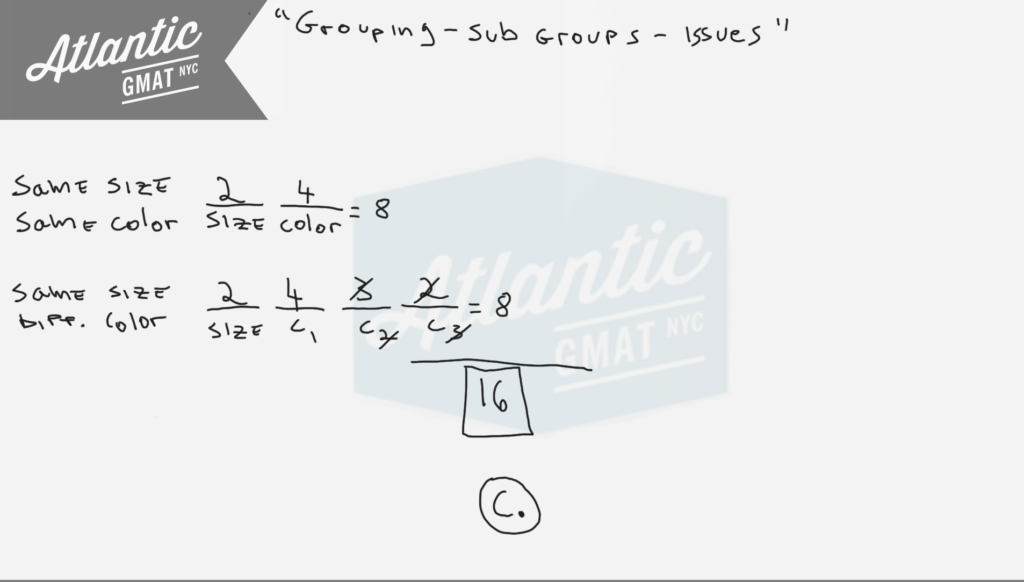In a certain city, 80 percent of the households have cable television, and 60 percent of the households have videocassette recorders. If there are 150,000 households in the city, then the number of households that have both cable television and videocassette recorders could be any number from:

(A) 30,000 to 90,000 inclusive
(B) 30,000 to 120,000 inclusive
(C) 60,000 to 90,000 inclusive
(D) 60,000 to 120,000 inclusive
(E) 90,000 to 120,000 inclusive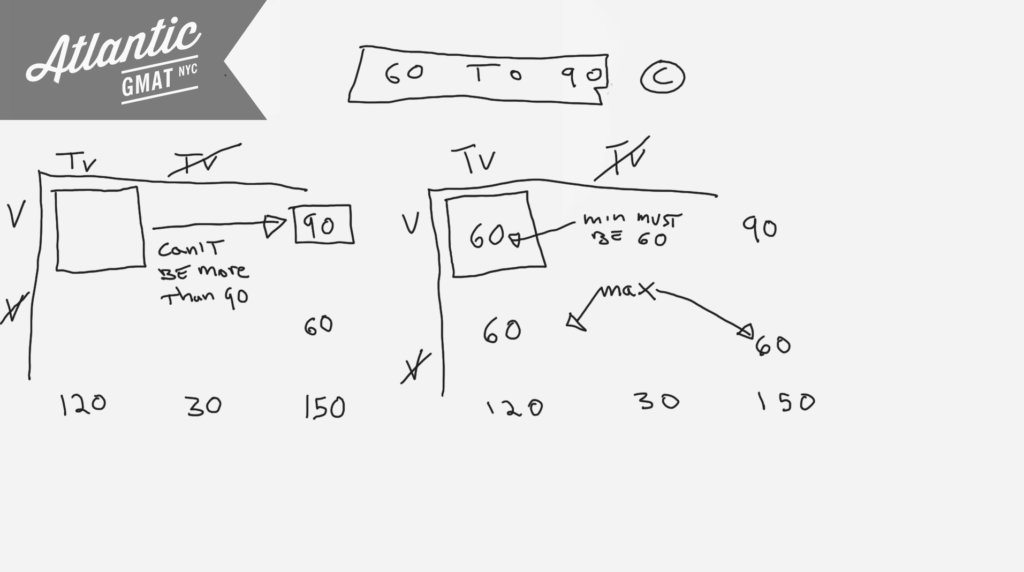For a finite sequence of non zero numbers, the number of variations in sign is defined as the number of pairs of consecutive terms of the sequence for which the product of the two consecutive terms is negative. What is the number of variations in sign for the sequence 1, -3, 2, 5, -4, -6 ?

(A) 1
(B) 2
(C) 3
(D) 4
(E) 5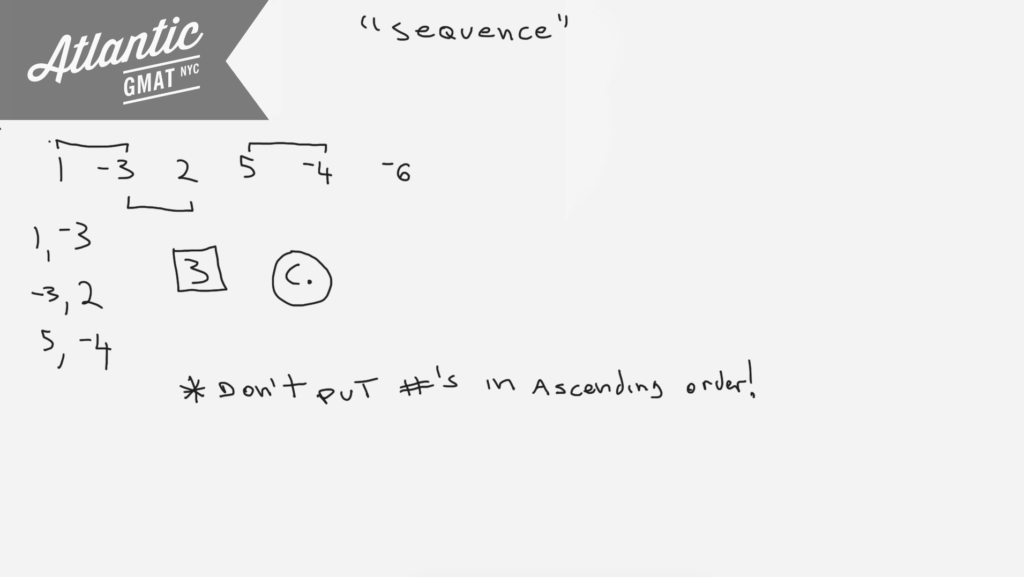SCORE INTERVAL----------------NUMBER OF SCORES

50-59-------------------------- 2
60-69--------------------------10
70-79--------------------------16
80-89--------------------------27
90-99--------------------------18

The table above shows the distribution of test scores for a group of management trainees. Which score interval contains the median of the 73 scores?

(A) 60-69
(B) 70-79
(C) 80-89
(D) 90-99
(E) It cannot be determined from the information given.

An investment of d dollars at k percent simple annual interest yields \$600 over a 2 year period. In terms of d, what dollar amount invested at the same rate will yield \$2,400 over a 3 year period?

(A) (2d)/3
(B) (3d)/4
(C) (4d)/3
(D) (3d)/2
(E) (8d)/3

A basket contains 5 apples, of which 1 is spoiled and the rest are good. If Henry is to select 2 apples from the basket simultaneously and at random, what is the possibility that the 2 apples selected will include the spoiled apple?

(A) 1/5
(B) 3/10
(C) 2/5
(D) 1/2
(E) 3/5

A small, rectangular park has a perimeter of 560 feet and a diagonal measurement of 200 feet. What is its area, in square feet?

(A) 19,200
(B) 19,600
(C) 20,000
(D) 20,400
(E) 20,800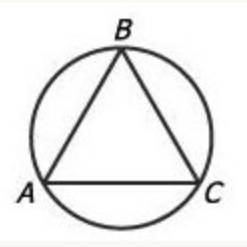In the figure above, equilateral triangle ABC is inscribed in the circle. If the length of arc ABC is 24, what is the approximate diameter of the circle?

(A) 5
(B) 8
(C) 11
(D) 15
(E) 19

It's key to know that an equilateral triangle has equal sides and equal angles measuring 60 degrees each. So each angle of an equilateral triangle inscribed in a circle cuts off 1/3 of the circle. Why is that? Review the basics! An angle on the circumference of a circle cuts off twice that angle's measure. So a 60 degree angle on the edge of a circle cuts off 120 degrees or 1/3 of a circle (120/360 degrees). A 60 degree angle in the center of a circle only cuts off 60 degrees (it doesn't have the extra space to open up). Examples below to illustrate this. Also, there's a special case for triangles that have the diameter of a circle as their base. Because the diameter is a 180 degree central angle, the angle opposite on the circumference of the circle must be 90 degrees. Not relevant for this question but good to know.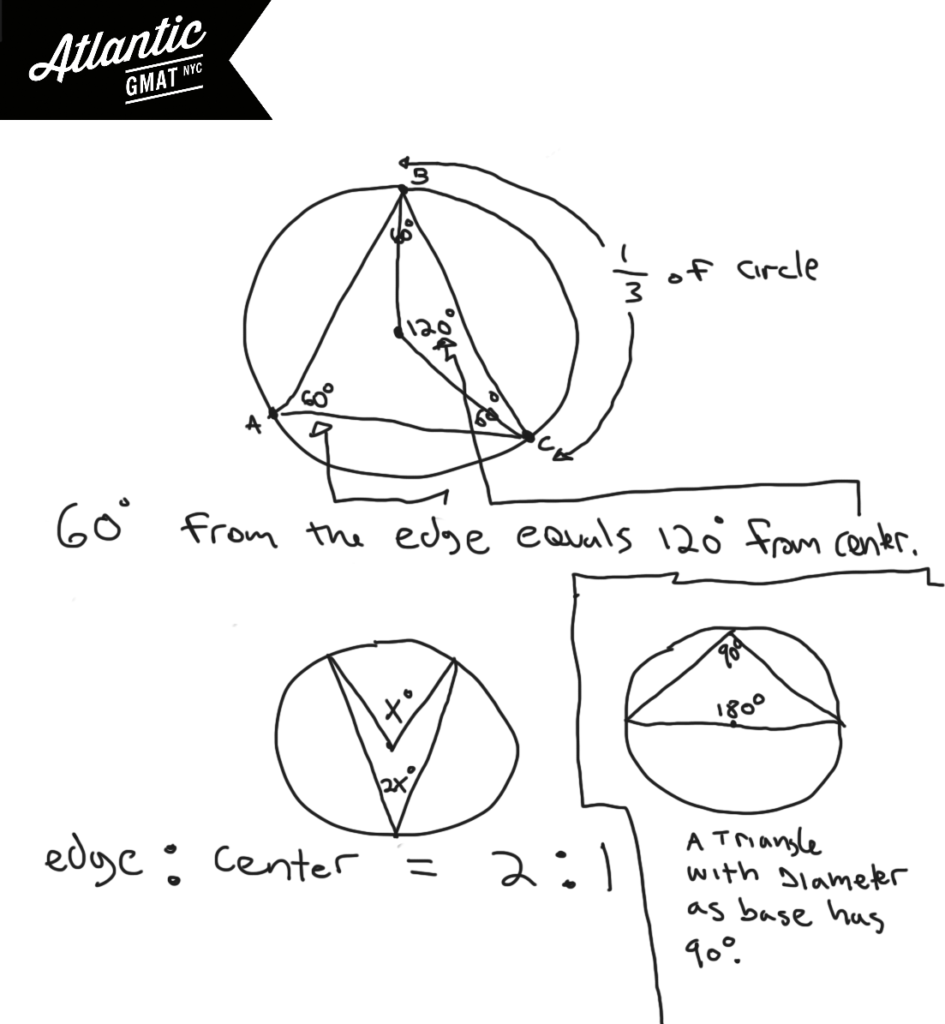How much of the circle is arc ABC? It's covering the same part of the circumference as two of the 60 degree angles from triangle ABA. Each of those angles represent 1/3 of the circle so arc ABC is 2/3 of the circle. If 2/3 is 24, 1/3 of the circle's circumference is 12, and the entire circumference is 36. So Pi*diameter = circumference. Pi*D = 36. D = 36/Pi. D = 36/3.14. A little less than 12. 11 is by far the closest answer choice. A little rusty feeling on these GMAT geometry rules? The rules are the easy part of GMAT studying. Get your fundamentals in shape ASAP. Avoid churning through questions before getting your basics GMAT quant organized. You don't want to waste good material and it's much harder to focus on the basics while working on tougher/trickier GMAT questions. Comment with any questions or additions. Happy studies!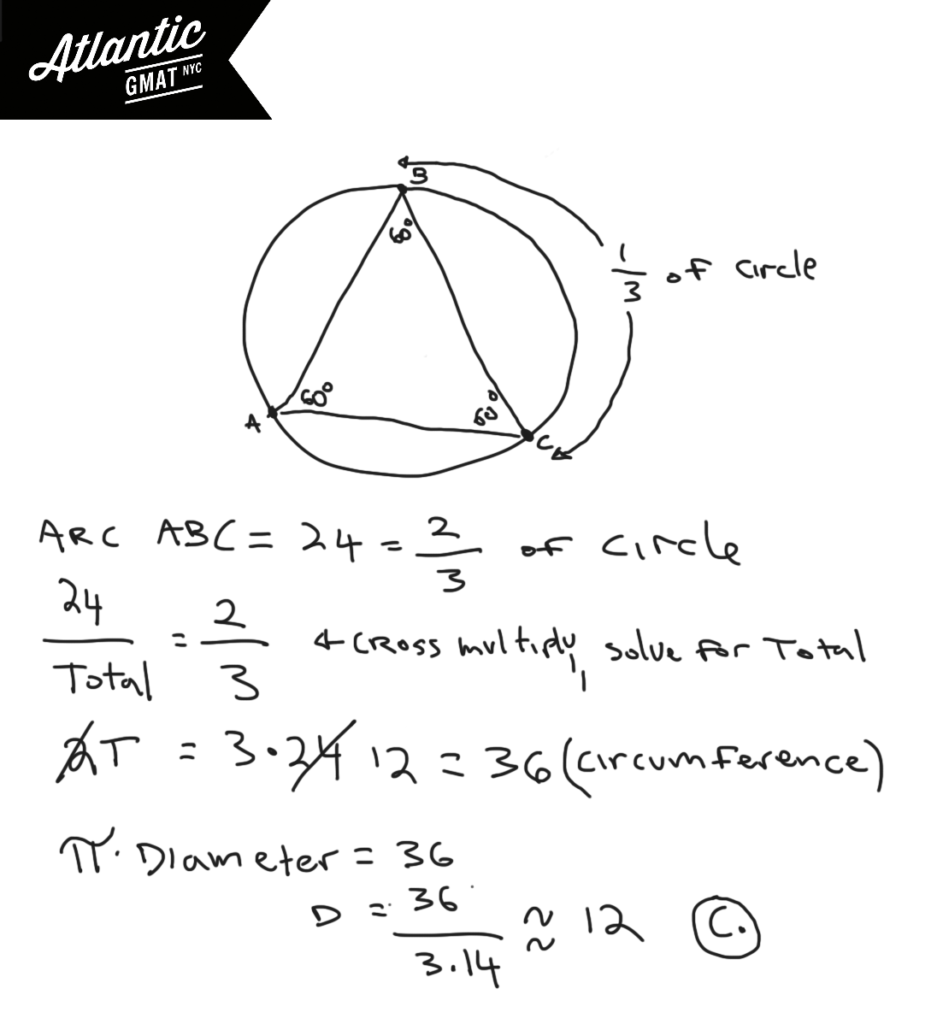A company has two types of machines, type R and type S. Operating at a constant rate, a machine of type R does a certain job in 36 hrs and a machine of type S does the same job in 18 hours. If the company used the same number of each type of machine to do the job in 2 hours, how many machines of type R were used?

(A) 3
(B) 4
(C) 6
(D) 9
(E) 12

This is from the free Official GMAT prep tests 1 and 2 so if you haven't done those yet: avoid this explanation. This is a work and rate question (cooperative rate). This specific type, adding a certain number of machines to get a job done faster, is not uncommon. I find the easiest way to approach is to get the cooperative rate of the machines by adding the rate of type R and type S. Why do we add? Well, they're working together on the same project. You subtract if they work against each other (you clean the apartment, your roommate messes it up). In this case the cooperative rate is 1/12. Put that in the T with a variable to count how many 1/12ths you need to get 1 job done in 2 hours. Here's a tougher version of this type of cooperative work and rate question. And here are a couple more similar questions from the GMAT prep software:

Official GMAT Work and Rate - Cooperative Rate 1

Official GMAT Work and Rate - Cooperative Rate 2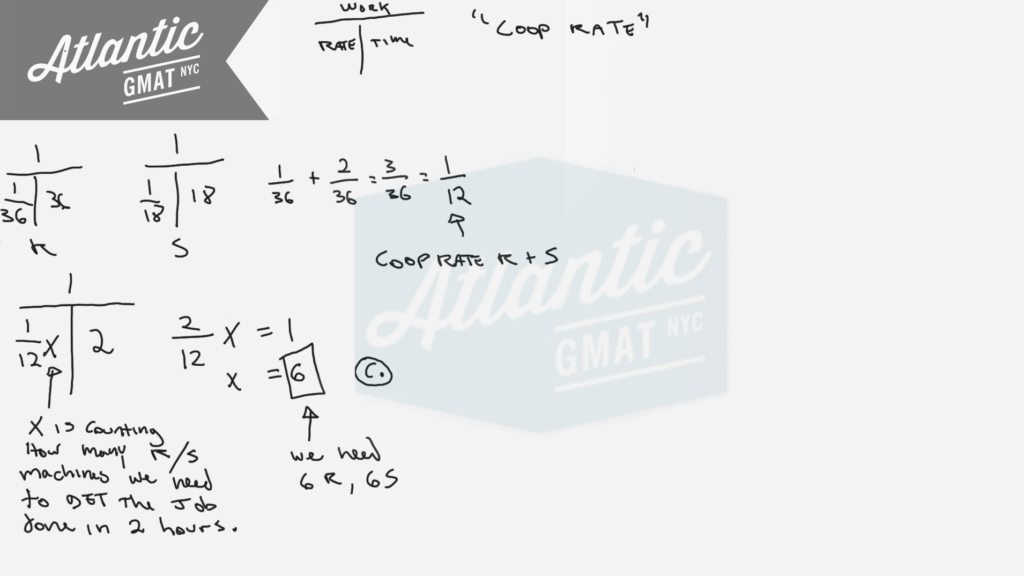Here's another set of cooperative work and rate questions to practice on:

Work and Rate 1

Work and Rate 2

Work and Rate 3

Work and Rate 4

Work and Rate 5

For more distance, work, and rate examples you can also check out our GMAT Question of the Day page

A right circular cone is inscribed in a hemisphere so that the base of the cone coincides with the base of the hemisphere. What is the ratio of the height of the cone to the radius of the hemisphere?

(A) √3:1
(B) 1:1
(C) 1/2/1
(D) √2:1
(E) 2:1

For a certain race, 3 teams were allowed to enter 3 members each. A team earned 6 – n points whenever one of its members finished in nth place, where 1 ≤ n ≤ 5. There were no ties, disqualifications, or withdrawals. If no team earned more than 6 points, what is the least possible score a team could have earned?

(A) 0
(B) 1
(C) 2
(D) 3
(E) 4

Here's another one from the GMAT Prep Software free tests 1 and 2 so better to wait on this explanation unless you've done those tests already. This is another GMAT puzzle that relies on you being organized. You basically need to make a list of the possibilities keeping track of the constraints with the goal of maximizing the points for two of the teams so the third team has the least possible score. In terms of the zoomed out approach this question reminds of this is other GMAT puzzle: The number 75 can be written as the sum of the squares of 3 different positive integers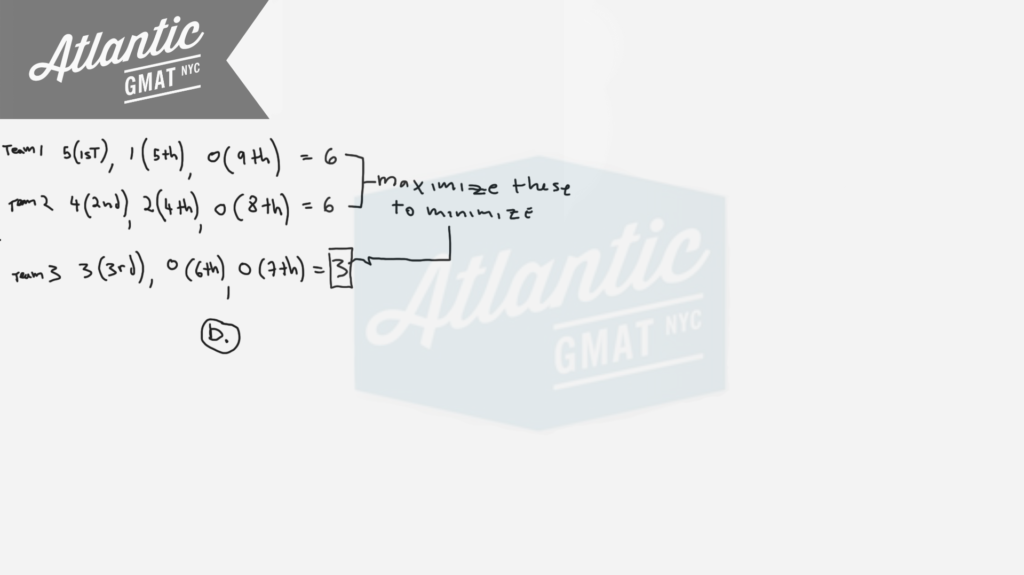A boat traveled upstream a distance of 90 miles at an average speed of (v-3) miles per hour and then traveled the same distance downstream at an average speed of (v+3) miles per hour. If the trip upstream took a half hour longer than the trip downstream, then how many hours did it take the boat to travel downstream?

(A) 2.5
(B) 2.4
(C) 2.3
(D) 2.2
(E) 2.1

This is a GMAT Average Rate question from the GMAT Prep tests. If you haven't yet done the GMAT Prep Tests then better to avoid solving this now so you have a fresh test experience. I'd organize this using the T's. Just put the information where it belongs. Make sure to define the question: How many hours did it take the boat to travel downstream? Basically we need to solve for V and then plug that value into the downstream T to get the time. There are two challenging things about this question that I see troubling GMAT tutoring students:

1. Often the "half hour longer" gets translated into 30 minutes. That causes chaos. If you use minutes then everything must be changed to that unit. I wouldn't suggest taking that route here.
2. Quadratics freak people out and cause panic which leads to poor decision making. Just because there's a quadratic doesn't mean that it's going to take a lot of work to solve. Slow down. Think about it for a second. Try to make a plan. In this case we know that the square must be between 30 and 40 (30*30 = 900, 40*40 = 1600) and must create a units digit of 9 limiting our options to 33 and 37. You might say: "but how do we know it's going to be a perfect square"??? Well, do you see radicals in the answer choices??? Nope. Try 33 first because 1089 is much closer to 900 than 1600. Take your time with the arithmetic. I like to use distribution math for multiplication.Here are more Official GMAT distance, work, and rate question for which we use the T method: Pumps A, B, and C operate at their respective constant rates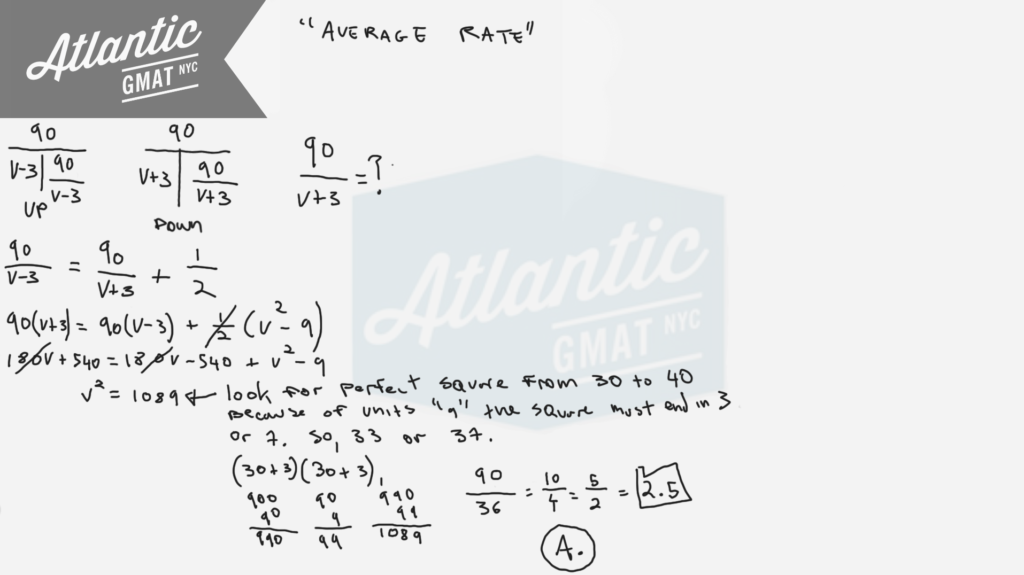Here's a bunch of extra practice questions focusing on average rates:

Distance, Work and Rate - Average Rate 1

Distance, Work and Rate - Average Rate 2

Distance, Work and Rate - Average Rate 3

For more GMAT distance, work, and rate practice visit GMAT Question of the Day.

For a certain exam, a score of 58 was 2 standard deviations below mean and a score of 98 was 3 standard deviations above mean. What was the mean score for the exam?

(A) 74
(B) 76
(C) 78
(D) 80
(E) 82

At a speed of 50 miles per hour, a certain car uses 1 gallon of gasoline every 30 miles. If the car starts with a full 12 gallon tank of gasoline and travels for 5 hours at 50 miles per hour, the amount of gasoline used would be what fraction of a full tank?

(A) 3/25
(B) 11/36
(C) 7/12
(D) 2/3
(E) 25/36

Last month 15 homes were sold in Town X. The average (arithmetic mean) sale price of the homes was \$150,000 and the median sale price was \$130,000. Which of the following statements must be true?

I. At least one of the homes was sold for more than \$165,000.
II. At least one of the homes was sold for more than \$130,0000 and less than \$150,000
III. At least one of the homes was sold for less than \$130,000.

(A) I only
(B) II only
(C) III only
(D) I and II
(E) I and III

A set of 15 different integers has median of 25 and a range of 25. What is greatest possible integer that could be in this set?

(A) 32
(B) 37
(C) 40
(D) 43
(E) 50

This GMAT question falls firmly into statistics. Foggy on median and range? Go back to the GMAT basics! Get this fundamental GMAT content down backwards and forwards so that you can focus on the critical thinking portion of the exam. Median is the middle number of an odd set or the average of the two middle numbers of an even set. Range is the biggest number minus the smallest number (Range = max - min). Let's start by plotting out what we know. Median is spatial so demands a diagram. In a set of 15 numbers the median is the 8th number (7 above it, 7 below it). Especially for statistics set questions avoid creating a million variables for numbers you're not solving for. Let's represent those numbers with dashes and create a variable for the min. Then you can add 25 to that for the max. Keep in mind what we're solving for: the greatest possible integer that could be in this set.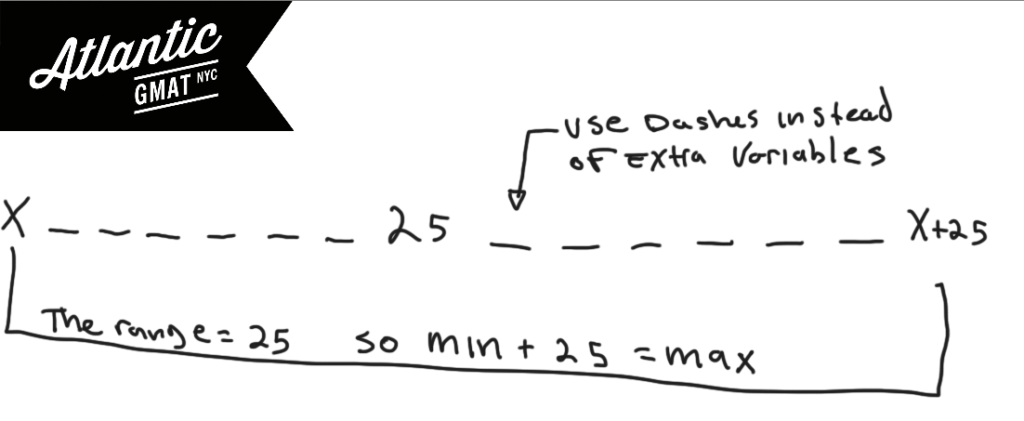We know the range is 25 and that we want to make the max as big as possible. So what should we do with the min? We want to make the min as big as possible so that min + 25 (the max) is as big as possible. You think: let's make the min = median. Good thinking! That would be perfect. But that's not possible as it is a set of 15 different integers. Still, we're on the right track. Let's just go down as little as possible from the median. 24.9999999999. Good thinking again! But, we have to stick with integers. So then you've got 24, 23, 22, 21, 20, 19, 18. Add 25 to 18 and that's the greatest possible integer in the set. Comment with any questions or additions. Happy GMAT studying!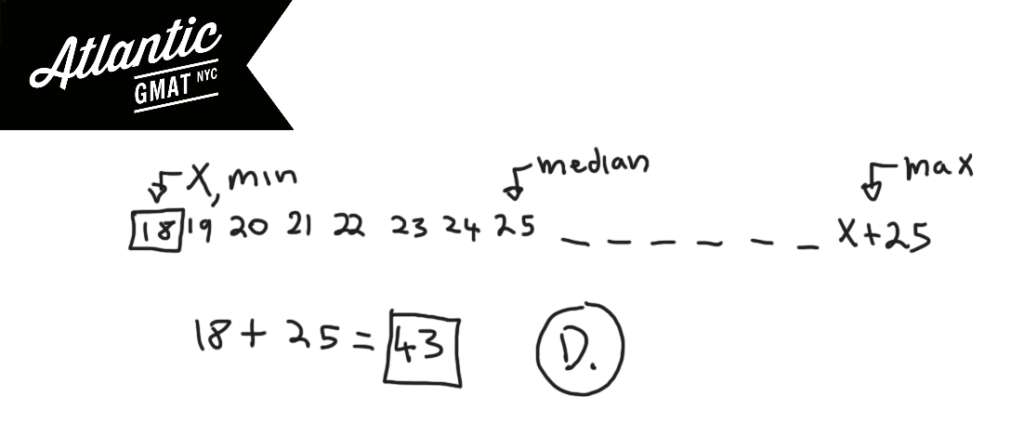A certain library assesses fines for overdue books as follows. On the first day that a book is overdue, the total fine is \$0.10. For each additional day that the book is overdue, the total fine is either increased by \$0.30 or doubled, whichever results in the lesser amount. What is the total for a book on the fourth day it is overdue?

(A) \$0.60
(B) \$0.70
(C) \$0.80
(D) \$0.90
(E) \$1.00

A survey was conducted to determine the popularity of 3 foods among students. The data collected from 75 students are summarized as below

48 like Pizza
45 like Hoagies
58 like tacos
28 like pizza and hoagies
37 like hoagies and tacos
40 like pizza and tacos
25 like all three food

What is the number of students who like none or only one of the foods?

(A) 4
(B) 16
(C) 17
(D) 20
(E) 23

The number of stamps that Kaye and Alberto had were in the ratio of 5:3 respectively. After Kaye gave Alberto 10 of her stamps, the ration of the number of Kaye had to the number of Alberto had was 7:5. As a result of the gift, Kaye had how many more stamps than Alberto?

(A) 20
(B) 30
(C) 40
(D) 60
(E) 90

In GMAT tutoring we teach the "ratio box". It's nothing groundbreaking but helpful for organizing GMAT ratio information. The Kaye and Alberto stamp question is a very specific type of ratio question that comes up reasonably often. I call it a "new ratio" question because usually you're given a new ratio and then asked to solve for some of the real world values. Let's take what we know about Kaye and Alberto and hopefully make some inferences. Here's the ratio box with the information that we have: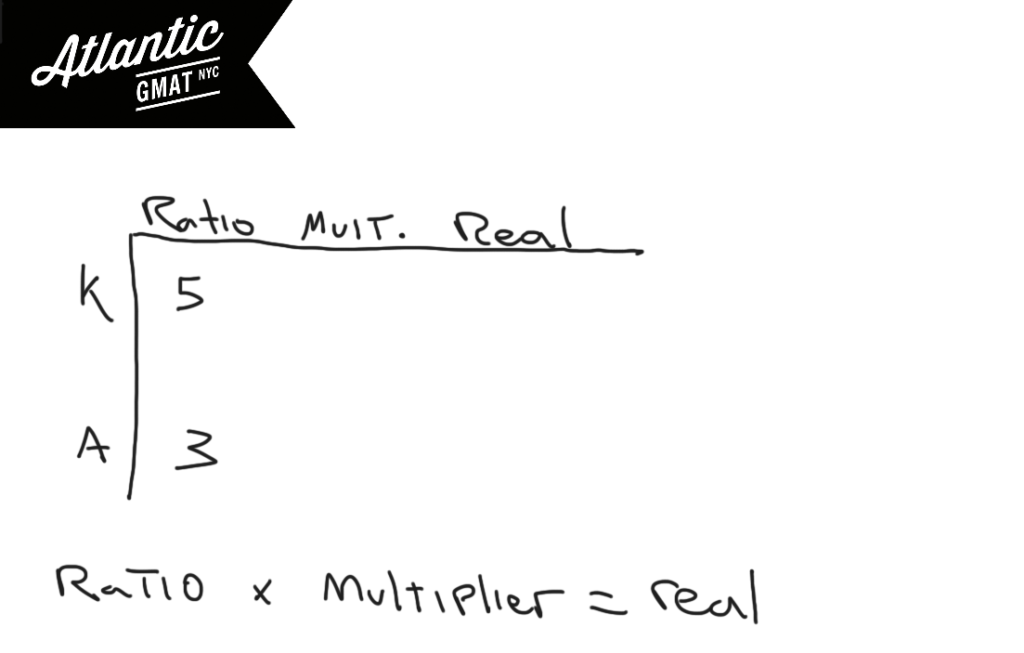We don't know what the multiplier is so I'd always put in a variable. I usually use "M" for multiplier. Using variables that somewhat connect to what they represent is a good GMAT habit to get into. Then you can multiply across to get your real world values for the stamps that Kaye and Alberto have. To those you can add 10 stamps to Alberto and subtract 10 stamps from Kaye. After the stamp trading, the new ratio of stamps is 7:5. Now we can just solve for the "M" the multiplier and then solve for the difference of stamps (after the trade). Go ahead and comment with any questions or additions. Happy GMAT studies!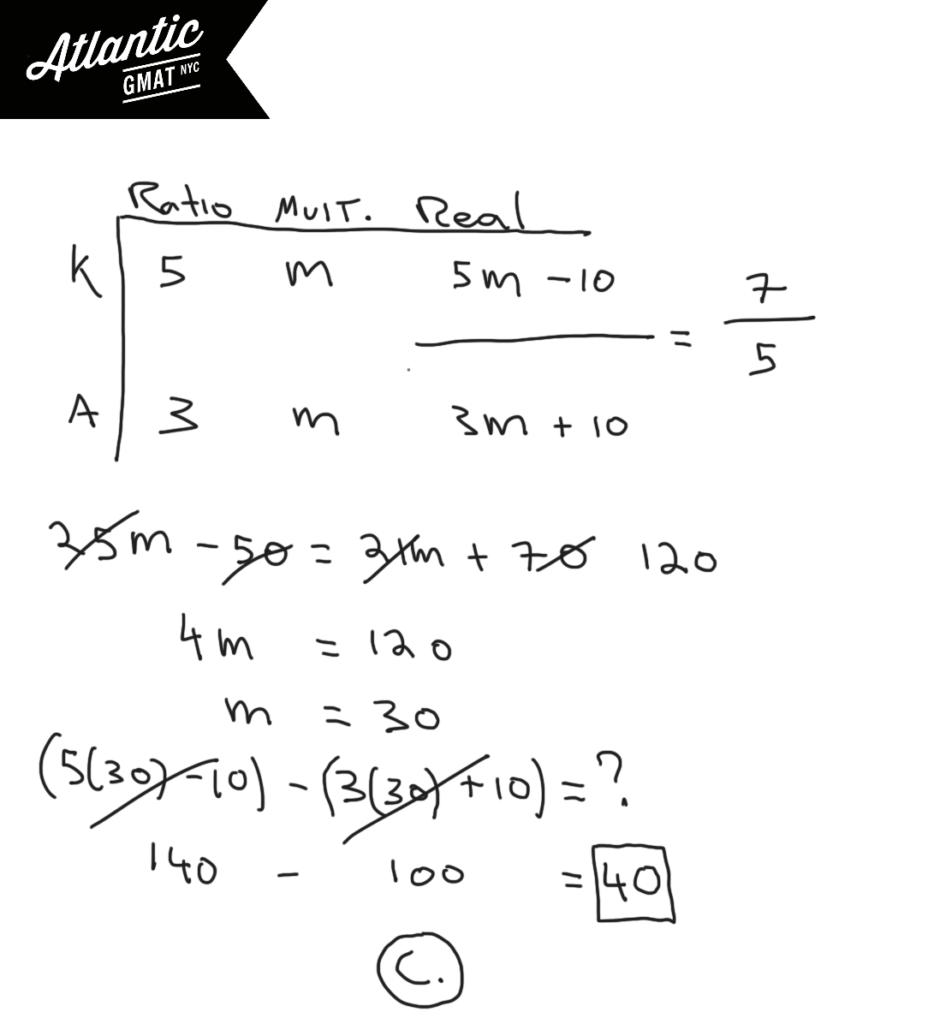The rate of a certain chemical reaction is directly proportional to the square of the concentration of chemical A present and inversely proportional to the concentration of chemical B present. If the concentration of chemical B is increased by 100 percent, which of the following is closest to the percent change in the concentration of chemical A required to keep the reaction rate unchanged?

(A) 100% decrease
(B) 50% decrease
(C) 40% decrease
(D) 40% increase
(E) 50% increase

7.51 8.22 7.86 8.36
8.09 7.83 8.30 8.01
7.73 8.25 7.96 8.53

A vending machine is designed to dispense 8 ounces of coffee into a cup. After a test that recorded the number of ounces of coffee in each of 1000 cups dispensed by the vending machine, the 12 listed amounts, in ounces, were selected from the data above. If the 1000 recorded amounts have a mean of 8.1 ounces and a standard deviation of 0.3 ounces, how many of the 12 listed amounts are within 1.5 standard deviation of the mean?

(A) Four
(B) Six
(C) Nine
(D) Ten
(E) Eleven

This question is from the the GMAT Prep Test 1 and 2 so if you haven't worked on those yet go ahead and skip this one for now. It's important to remember that standard deviation is plus or minus. So 1 standard deviation out would be 8.4 on the upper end and 7.8 on the lower end. 1.5 standard deviations out is 8.55 on the upper end and 7.65 on the lower end. Now you just have knock out amounts out of that range. Working backwards is faster since it becomes clear that most of the measurements are falling within 1.5 standard deviations of the mean. Let's also remember that we're not basing all of this on the 8 ounces the vending machine was designed to dispense but on the 8.1 mean that was calculated based on the 1000 cups actually dispensed.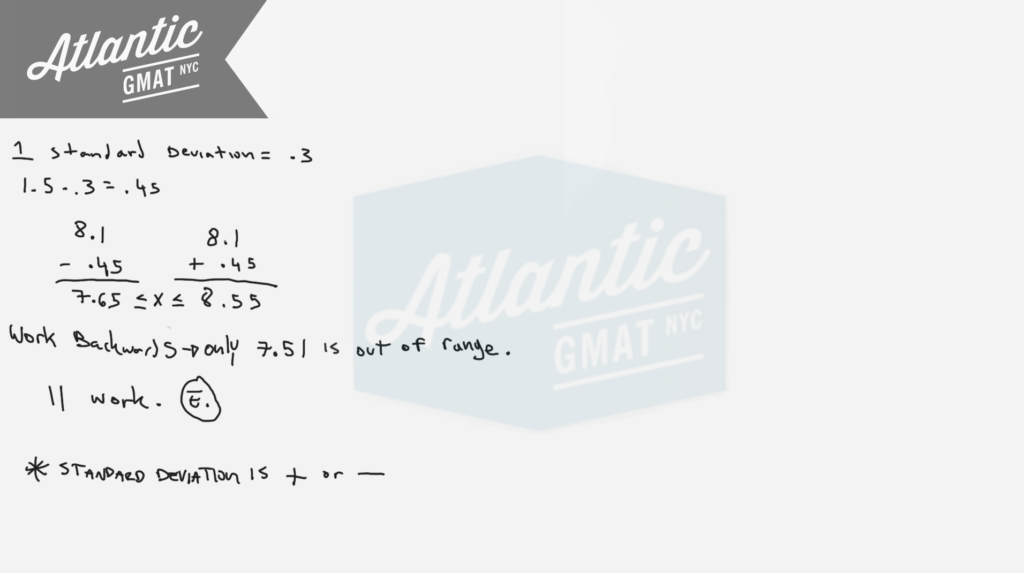The number 75 can be written as the sum of the squares of 3 different positive integers. What is the sum of these 3 integers?

(A) 17
(B) 16
(C) 15
(D) 14
(E) 13

This question is from the free official GMAT practice tests 1 and 2 so avoid if you haven't done those already. This is a number properties puzzle that benefits from doing a bit of organizing. The sum of the squares of the 3 integers sum is 75. So go ahead and list the first bunch of squares starting from 1 up to just below 75. Once you've got that you just need to find the combination that works. To make your job easier use units digits. 75 ends in 5 so your three numbers must create that units digit. The other tip is: start from the bigger integers and work down. To get to 75 you're going to need one of those big guys (64, 49, or 36). Finding a constraint can be very helpful (in this case the units digit and the requirement of 36, 49, or 64). Usually with these GMAT puzzles there are little shortcuts. Generally you don't pull them out of thin air. They are the natural conclusion of good organization. Make sure to take your time with setup so you pave the way for success. Here's another GMAT question that relies on just staying organized and paying attention: For a certain race, 3 teams were allowed to enter 3 members each. A team earned 6 – n points whenever one of its members finished in nth place, where 1 ≤ n ≤ 5. The details are very different but the style is similar and I think it requires the same kind of thinking.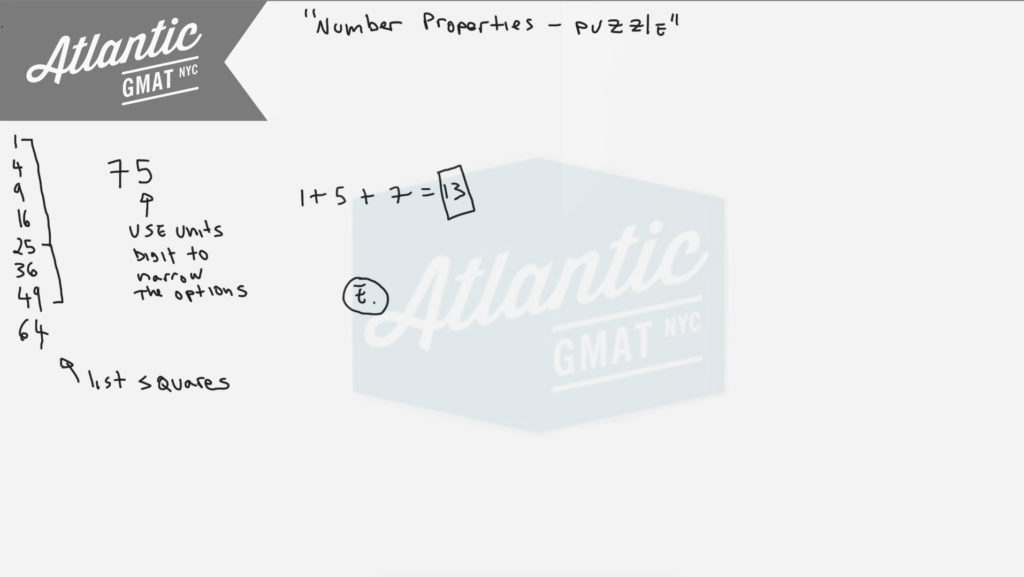If M = √4 + 4^1/3 + 4^1/4, then the value of M is:

(A) Less than 3
(B) Equal to 3
(C) Between 3 and 4
(D) Equal to 4
(E) Greater than 4

If xy + z = x(y + z), which of the following must be true?

(A) x = 0 and y = 0
(B) x = 1 and y = 1
(C) y = 1 and z = 0
(D) x = 1 or y = 0
(E) x = 1 or z = 0

In a village of 100 households, 75 have at least one DVD player, 80 have at least one cell phone, and 55 have at least one MP3 player. If x and y are respectively the greatest and lowest possible number of households that have all three of these devices, x – y is:

(A) 65
(B) 55
(C) 45
(D) 35
(E) 25

Pumps A, B, and C operate at their respective constant rates. Pumps A and B, operating simultaneously, can fill a certain tank in 6/5 hours; pumps A and C, operating simultaneously, can fill the tank in 3/2 hours; and pumps B and C, operating simultaneously, can fill the tank in 2 hours. How many hours does it take pumps A, B, and C, operating simultaneously, to fill the tank.

(A) 1/3
(B) 1/2
(C) 1/4
(D) 1
(E) 5/6

This is a cooperative rate question so I would recommend using the T's to organize the information. It's a word problem so take your time reading and taking inventory of the question. Initially don't worry too much about the numbers. Pay attention to the structure and the question itself which you should define before doing any calculations. My first move is to splash out three T's to represent the three rates that were given, A + B, A + B, and B + C. We know that the rates need to be added because the pumps are operating simultaneously to fill the tank. What do we want to calculate: How many hours does it take pumps A, B, and C, operating simultaneously, to fill the tank? So we want to solve for the cooperative rate A + B + C  and then with that calculate the time it takes to get the tank filled. So, go ahead and add up the three sets of cooperative rates (5/6, 2/3, 1/2) and set them equal to the three cooperative rates they represent (A + B, A + C, B + C). Then group like terms and get common denominators for the fractions. You should end up with 2(A + B + C) = 2 or A + B + C = 1. The rate of 1 tank per hour means that the time it takes to fill the tank is, you guessed it: 1 hour.

Here are more Official GMAT distance, work, and rate question for which we use the T method:

Circular gears P and Q start rotating at the same time at constant speeds

A boat traveled upstream a distance of 90 miles at an average speed of (v-3) miles per hour and then traveled the same distance downstream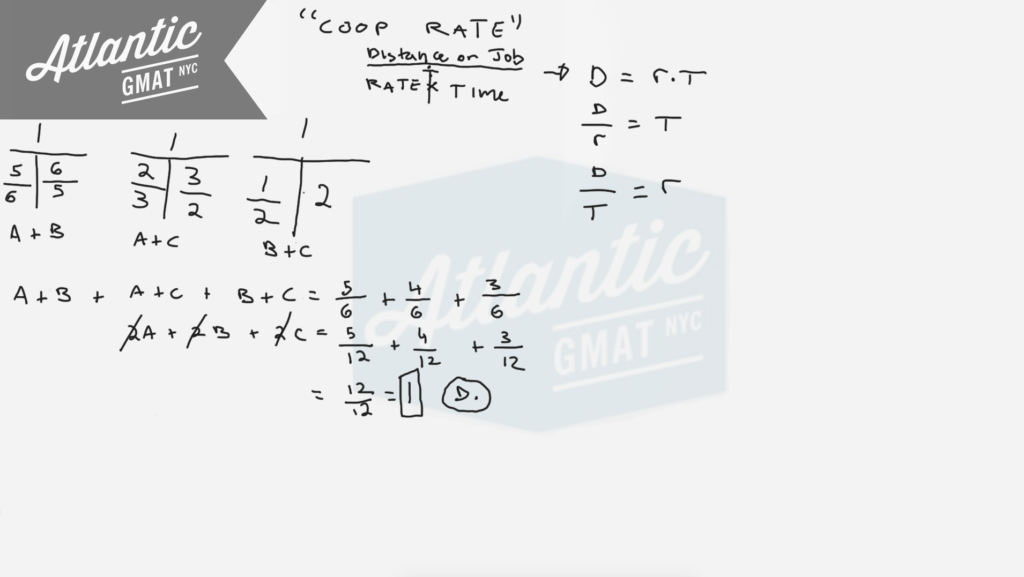Here are another bunch of cooperative work and rate questions to practice on:

Work and Rate 1

Work and Rate 2

Work and Rate 3

Work and Rate 4

Work and Rate 5

For more distance, work, and rate examples you can also check out our GMAT Question of the Day page

Running at their respective constant rates, machine X takes 2 days longer to produce w widgets than machines Y. At these rates, if the two machines together produce 5w/4 widgets in 3 days, how many days would it take machine X alone to produce 2w widgets.

(A) 4
(B) 6
(C) 8
(D) 10
(E) 12

When a certain tree was first planted, it was 4 feet tall and the height of the tree increased by a constant amount each year for the next 6 years. At the end of 6 year the tree was 1/5 taller than it was at the end of 4 year. By how many feet did the height of the tree increase each year

(A) 3/10
(B) 2/5
(C) 1/2
(D) 2/3
(E) 6/5

The "tree" questions comes up about 50% of the time in GMAT tutoring sessions. There's nothing crazy going on here but you do need to focus on the question and then stay organized in the follow through. I'd go ahead and draw a little chart to make sense of the information. When the tree was first planted it was 4 feet tall so that's the start of the number line and then it grew by a constant amount each year so assign a variable for that, say x. Then you can define the 6th year in terms of the 4th year (the 6th year is 5/4ths the 4th year). That's it. Solve for x.

Alice’s take-home pay last year was the same each month, and she saved the same fraction of her take-home pay each month. The total amount of money that she had saved at the end of the year was 3 times the amount of that portion of her monthly take-home pay that she did NOT save. If all the money that she saved last year was from her take-home pay, what fraction of her take-home pay did she save each month?

(A) 1/2
(B) 1/3
(C) 1/4
(D) 1/5
(E) 1/6

A used car dealer sold one car at a profit of 25 percent of the dealers purchase price for that car and sold another car at a loss of 20 percent of the dealers purchase price for that car. If the dealer sold each car for \$20,000, what was the dealers total profit or loss, in dollars, for the two transactions combined?

(A) \$1,000 profit
(B) \$2,000 profit
(C) \$1,000 loss
(D) \$2,000 loss
(E) \$,3334 loss

If the operation @ is defined for all integers a and b by a@b = a+b - ab, which of the following statements must be true for all integers a, b and c?

I. a@b = b@a
II. a@0 = a
III. (a@b)@c = a@(b@c)

(A) I only
(B) II only
(C) I and II only
(D) I and III only
(E) I, II and III

Circular gears P and Q start rotating at the same time at constant speeds. Gear P makes 10 revolutions per minute and Gear Q makes 40 revolutions per minute. How many seconds after the gears start rotating will gear Q have made exactly 6 more revolutions than gear P?

(A) 6
(B) 8
(C) 10
(D) 12
(E) 15

Another question from the GMAT Prep software so avoid if you haven't yet worked through the free official practice tests so that you can keep those fresh. There's more work and rate practice below that you can safely work through now. This is technically a work and rate cooperative rate question even though it doesn't seem like it. I'd still use the T's to organize the information but then I'd probably move to algebraic translation. After setting up the first T's go ahead and do the unit conversion. We have to convert to seconds so multiply your minutes by 60 and then do the arithmetic for the rest of the chart. From there we need to figure out: How many seconds after the gears start rotating will gear Q have made exactly 6 more revolutions than gear P? So the seconds (S) in my equation are the same for gears P and Q because the gears are rotating at the same time. The only difference is that we need to add 6 revolutions to P's side the equation (to balance out the fact that Q will have made exactly 6 more revolutions than P).

Here are more Official GMAT distance, work, and rate question for which we use the T method:

Pumps A, B, and C operate at their respective constant rates

A company has two types of machines, type R and type S

A boat traveled upstream 90 miles at an average speed of (v-3) miles per hour and then traveled the same distance downstream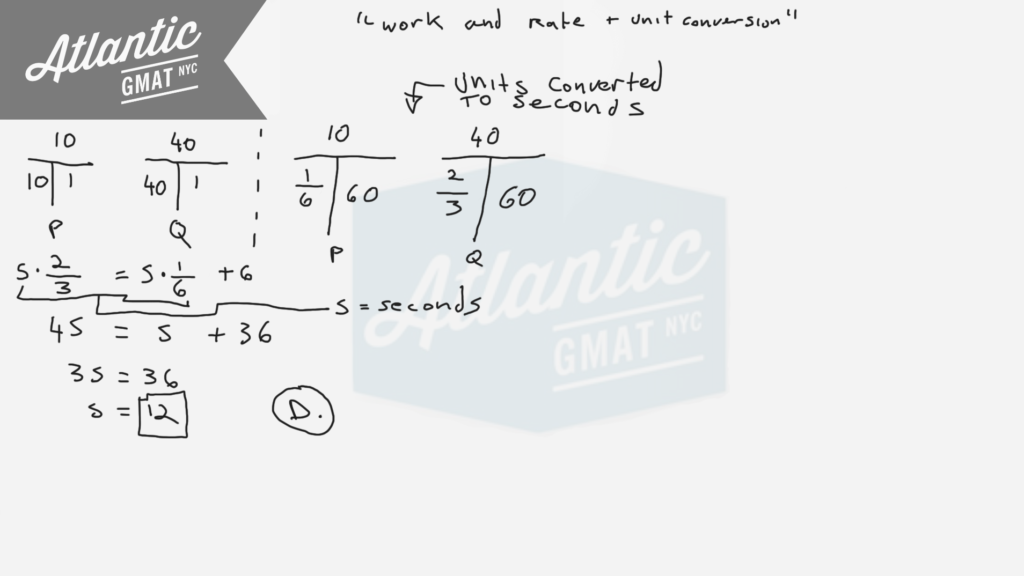Here's another set of cooperative work and rate questions to practice on:

Work and Rate 1

Work and Rate 2

Work and Rate 3

Work and Rate 4

Work and Rate 5

For more distance, work, and rate examples you can also check out our GMAT Question of the Day page

Three grades of milk are 1 percent, 2 percent and 3 percent fat by volume. If x gallons of the 1 percent grade, y gallons of the 2 percent grade, and z gallons of the 3 percent grade are mixed to give x+y+z gallons of a 1.5 percent grade, what is x in terms of y and z?

(A) y + 3z
(B) (y  + z)/4
(C) 2y + 3z
(D) 3y + z
(E) 3y + 4.5z

If n denotes a number to the left of 0 on the number line such that the square of n is less than 1/100, then the reciprocal of n must be

(A) Less than -10
(B) Between -1 and -1/10
(C) Between -1/10 and 0
(D) Between 0 and 1/10
(E) Greater than 10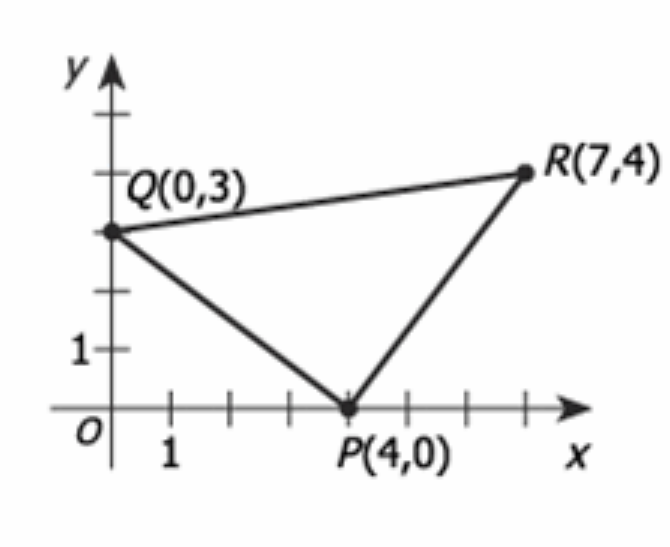In the rectangular coordinate system above, the area of triangular region PQR is

(A) 12.5

(B) 14

(C) 10√2

(D) 16

(E) 25

For GMAT geometry questions be active with diagrams. Don't be satisfied with what you're given. Try to make simple additions that provide more information (inferences). In general when trying to find an area on the coordinate plane you need to make a square, rectangle, or at the least, a right triangle. Right angles make measurement possible. Also, be open to the idea of adding/subtracting the areas of two or more shapes in order to solve for the desired area. In this case I'd draw two extra lines to make a rectangle.With that you should notice that you've created extra triangles and that those are right triangles. On GMAT geometry right triangles are your friends because you can do Pythagorean theorem and calculating are uses on the sides of the triangle (there's no need to drop an altitude). Now you can calculate the area of the rectangle and then subtract from that the areas of the three right triangles. That will leave the area of the middle triangle. Comment with any question or additions. Happy studies!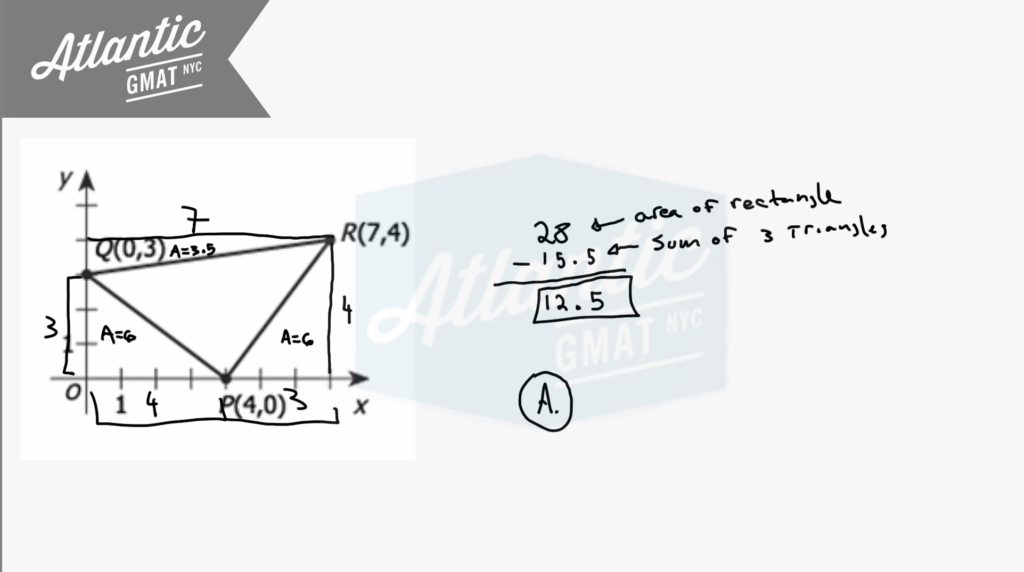According to the directions on a can of frozen orange juice concentrate, 1 can of concentrate is to be mixed with 3 cans of water to make an orange juice. How many 12 ounce cans of concentrate are required to prepare 200 6-ounce servings of orange juice?

(A) 25

(B) 34

(C) 50

(D) 67

(E) 100

It's almost always the case that GMAT tutoring students miss that this is just a ratio. You're making a solution (the orange juice) that has a certain mix of different ingredients (water and concentrate) that are in a certain proportion. That screams: ratio! For GMAT ratio questions I like making a ratio box to organize the information. You don't always have to use it but often the ratio box is a helpful tool. On the top of the box you've got your Ratio, Multiplier, and Real. You multiply the ratio by the multiplier to get to the real world values. The multiplier is always the same all the way down. Notice that you can sum your Ratio column to the get the ratio total. Same for the Real. You can't sum the multiplier column.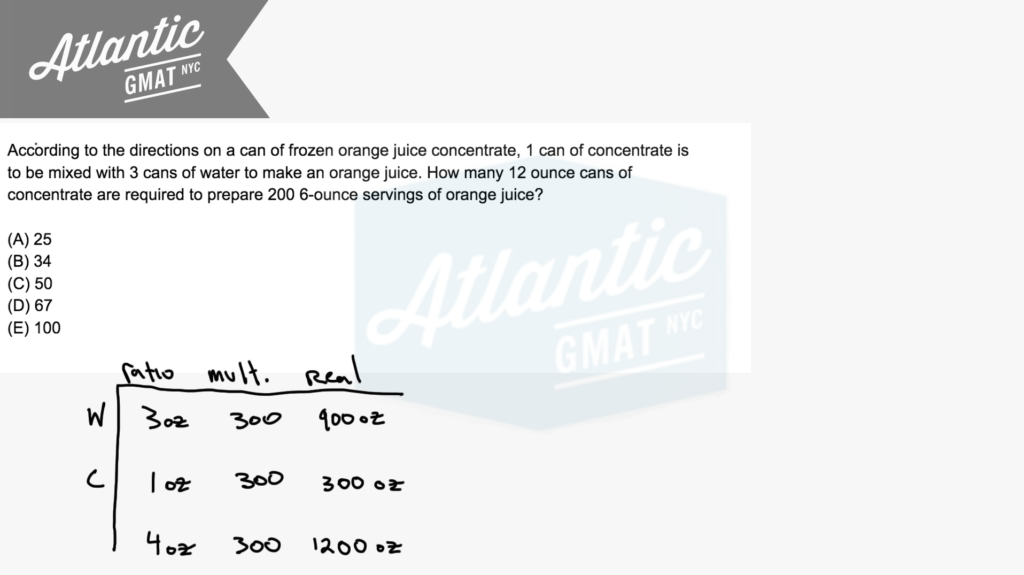Now that we've got our information in the ratio box hopefully we can make some inferences. In total we've got 1200 ounces of orange juice. For that 1200 ounces we need 300 ounces of concentrate. The cans have 12 ounces of concentrate. So 300/12 = 25. Done. Comment with any questions or additions!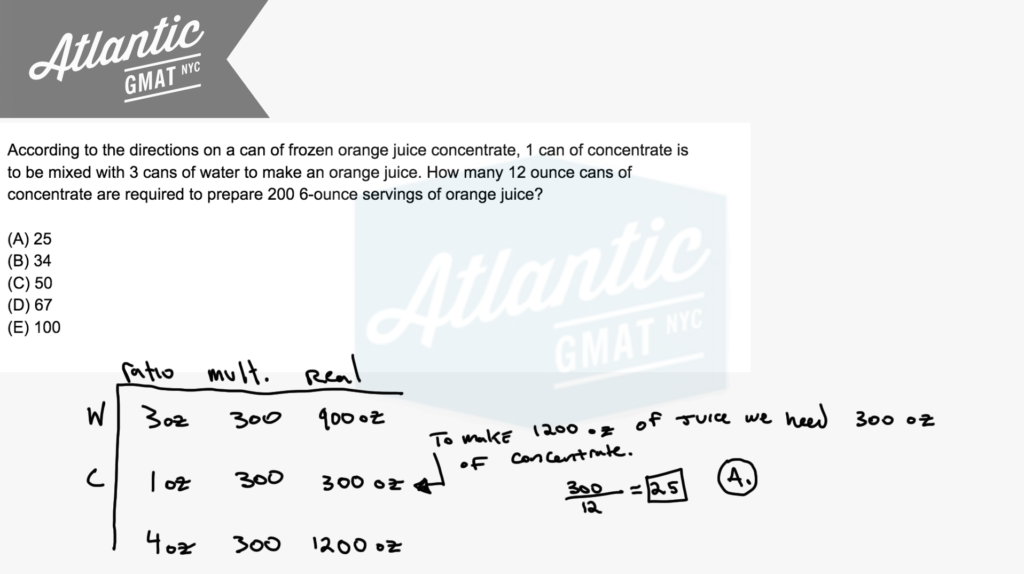If two of the four expressions x+y, x+5y, x-y, and 5x-y are chosen at random, what is the probability that their product will be of the form of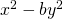, where b is an integer?

(A) 1/2

(B) 1/3

(C) 1/4

(D) 1/5

(E) 1/6

Be super comfortable with difference of squares. You're almost guaranteed to need it on your GMAT. You need to know it forwards, backwards, and upside-down. There isn't much content you need to know for the GMAT quant but in order to be consistently successful so that you can go in there on test day and snag your 700+ score you need complete fluency with that limited content. On this one we're looking for a product of two terms that will produce difference of squares. So we need something in the format: (x+y(x-y). There's only one pairing that gives us the right format. Now you have to figure out what that means in terms of probability. In general, probability is specific scenario/total scenarios. You can calculate the total and the specific scenarios using the slot method. How many teams of 2 can you make from four things? (4*3)/(1*2) = 6. So that's your denominator. And then there's only one team that works, the pairing of (x + y)(x - y). So that leaves you with 1/6. E. Detailed diagrams below and an in depth video explantation here: If two of the four expressions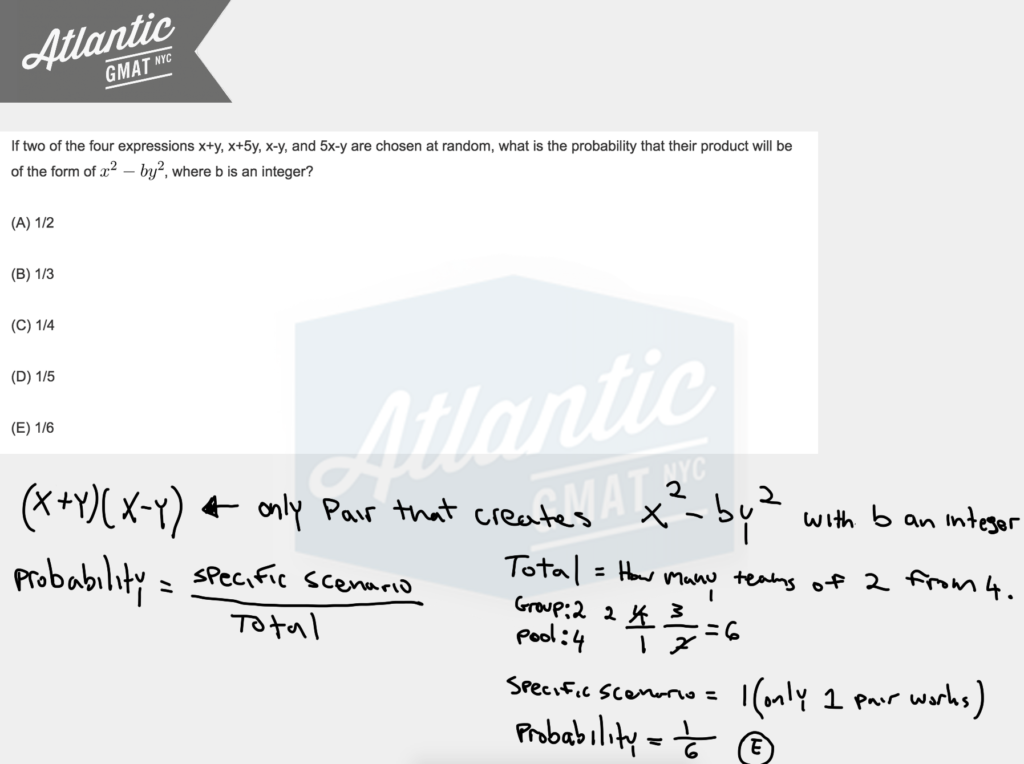You also can think about this in terms of straight probability and say: 2 choices our of 4 and then 1 choice out of 3. 2/4 * 1/3 = 2/12 = 1/6. Comment with any questions or additions. Happy studies!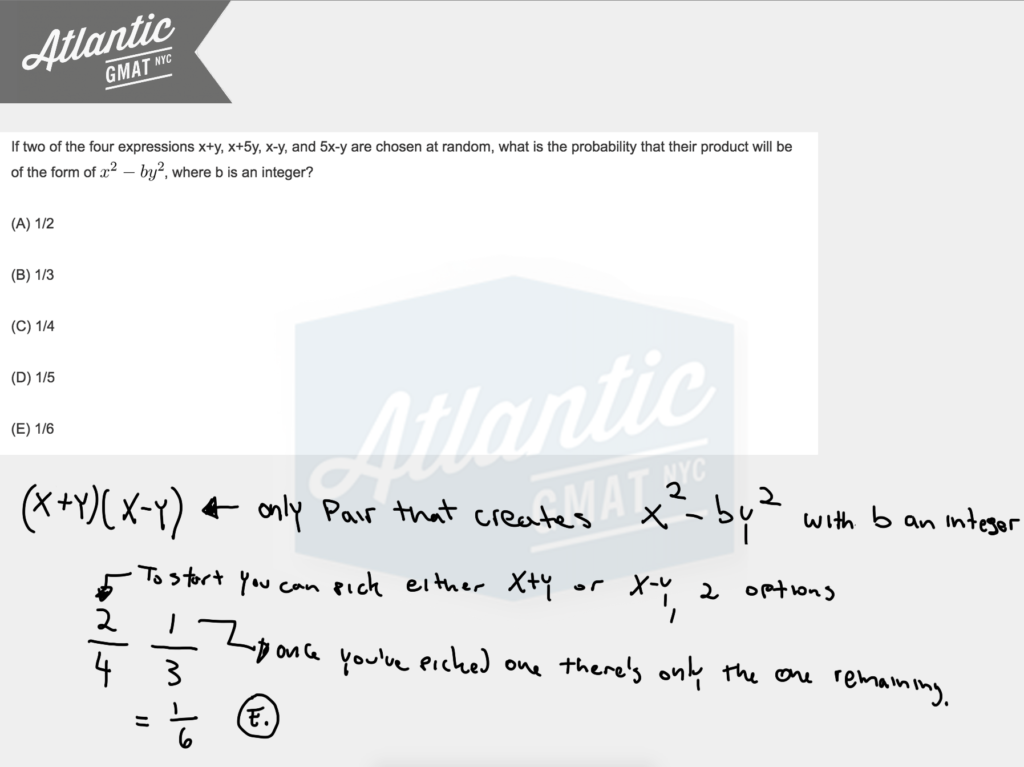Which of the following lists the number of points at which a circle can intersect a triangle?

(A) 2 and 6 only

(B) 2, 4 and 6 only

(C) 1, 2, 3 and 6 only

(D) 1, 2, 3, 4 and 6 only

(E) 1, 2, 3, 4, 5 and 6 only

I'm often asked: "What's the most important thing to work on in order to succeed on the GMAT". Not a simple question. Of course you need all of the quant and verbal fundamentals. And you're going to need to learn GMAT specific strategy. Above all that though is what I think is the most important quality: organized curiosity. You have to be willing to explore a bit. Not randomly though. In an organized way. Conducting mini experiments to feel out a question. This geometry question from the GMAT prep tests is a good example of the type of GMAT puzzle that rewards those willing to try a few things. Most often students stare at this one and refuse to play a little bit drawing out scenarios. Time pressure gets the best of them and then it's over. You have to ignore the clock a bit so you can let some ideas flow. GMAT time pressure is real. In that it's a timed exam and for most people that adds a formidable constraint. Still, if you're going to spend precious time working on a question better make that quality time leaving yourself open to the type of thinking that tends to lead to success. Developing the habit of organized curiosity requires taking the risk of ignoring the clock. Hopefully you'll find that by opening things up a bit you think more flexibility and creatively and give yourself the time to organize things properly so that you actually are not only more effective but more efficient. This isn't a skill that you just turn on. It needs to be practiced. And while you're practicing you're probably going to fail a whole bunch. But remember to keep practicing how you actually want to perform. It's true that in the short term you might be able to get away with lower quality thinking and make some gains refining that but if you're looking to fundamentally change your GMAT performance you likely need to not only work on GMAT content by your general approach to problem solving. Diagram below with the solution and here's a video explanation: Which of the following lists the number of points at which a circle can intersect a triangle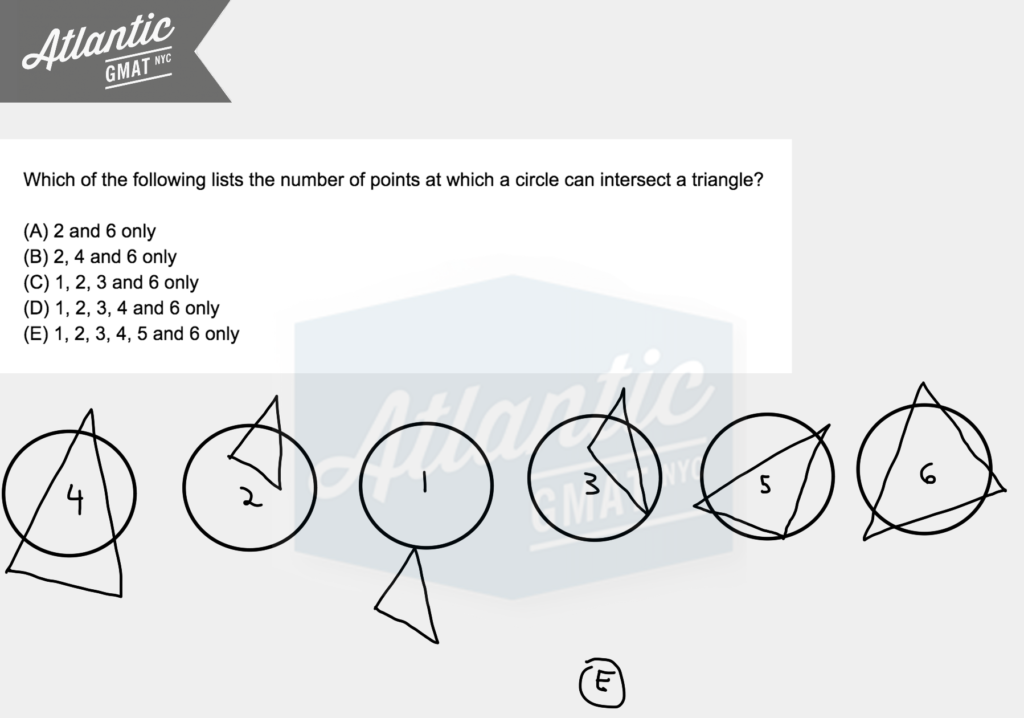A committee of 3 people is to be chosen from four married couples. What is the number of different committees that can be chosen if two people who are married to each other cannot both serve on the committee?

A. 16
B. 24
C. 26
D. 30
E. 32

GMAT combinatorics questions get demonized but they are among the most formulaic to tackle. Are there tough ones? Yep. As there are though questions related to any type of GMAT content. But on the whole they're not tougher than anything else and tend to require little calculation. So if you know the system most GMAT combinatorics questions don't take a lot of time or effort. Usually the first thing to think about is whether you're dealing with a group or an order. Are you picking friends to go on vacation with you or are you thinking about how many ways you can line people up? Creating committee's of three people sounds like ordering. There's no spatial element. It's just picking a certain number of people from a larger pool. In this one we're picking three people from a group of 8. However there's a constraint! Always double check for constraints. You don't always have them but often you do. In this case married people can't be together. So you have 8 people but limitations on who can be grouped. Not a big deal. We just have to consider it. Here at Atlantic GMAT we tackle grouping questions as follows:

The group defines the number of slots you create. The pool is the number of people that can go into the slots. See digram below and/or check out the video solution: a committee of 3 people is to be chosen from four married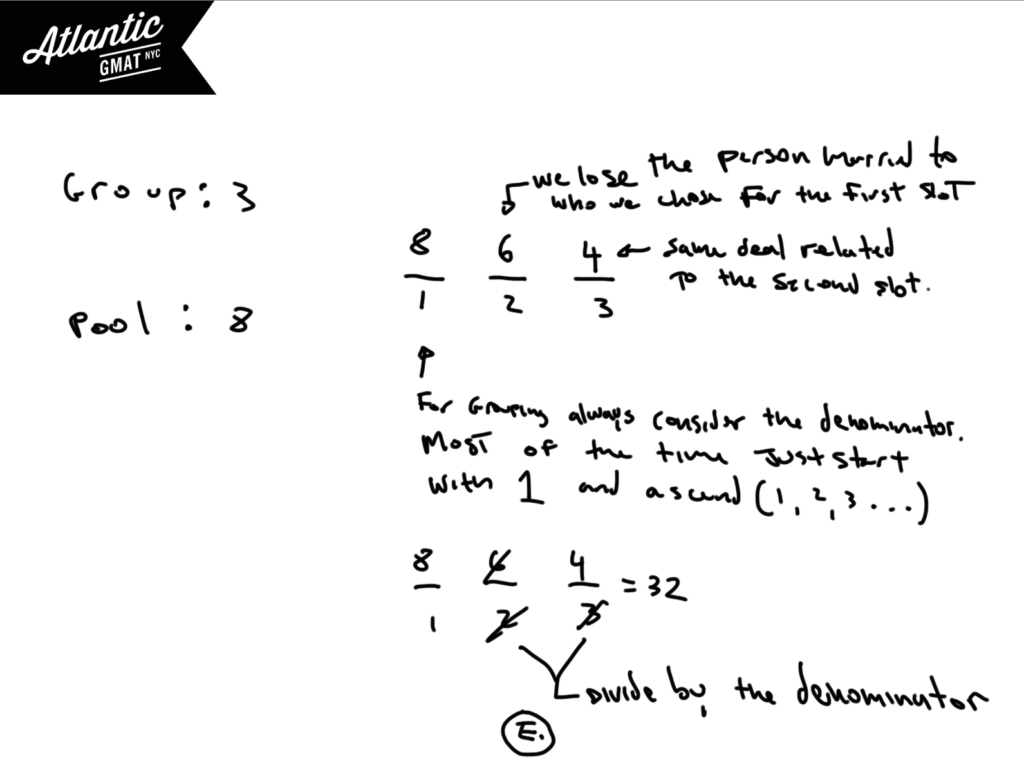The biggest point of confusion is the denominator. Why is it numbered? Why do we divide? You almost always divide when doing grouping, Why? If you don't then you over-count. Why? Because then you are counting the spatial element. If you don't divide then group A, B, C is also counted as C, B, A and B, C, A, and B, A, C even though those are all the same group. Another way to look at the division is that you're dividing by the number of times that three things can be ordered: 3!. That way you don't count the spatial/ordering. Here are some extra combinatorics questions to practice on: GMAT Question of the day Combinatorics. These are not official GMAT questions and in general are very difficult. That said, there's a lot to learn from them in terms of combinatorics technique. Comment with any questions or additions. Happy studies!

The perimeter of a certain isosceles right triangle is 16 + 16√2. What is the length of the hypotenuse of the triangle?

(A) 8

(B) 16

(C) 4√2

(D) 8√2

(E) 16√2

A lot of people get this GMAT geometry question from the GMAT prep software wrong. It's true that the algebra gets a little tricky but conceptually there's not much going on. And, to add, there are three ways to solve this thing. You can tackle the algebra, plug in answer choices, or you can even estimate. We're going to take a look at each solution. Let's start with the algebraic one. You need to know that the sides of an isosceles right triangle are always in the ratio x, x, x√2. No excuses for not knowing GMAT basics. You have to be fluent with the fundamentals so that you can focus on what the test is really about: critical thinking. Back to the solution! The perimeter then is 2x + x√2. And that equals 16 + 16√2. About half of people stop here and stay: Bingo! The hypotenuse must be 16√2!!! Nope. That's some fuzzy logic. Let's keep simplifying by trying to isolate the variable. You can do that by factoring out the x. Do you know how to get rid of radicals in the denominator (rationalizing)? Well, that's another basic that you'll need to conquer the GMAT quant. Keep in mind that we're solving for x√2 (the hypotenuse). See the diagram below for the worked out solution and/or check out the video solution here: the perimeter of a certain isosceles right triangle is 16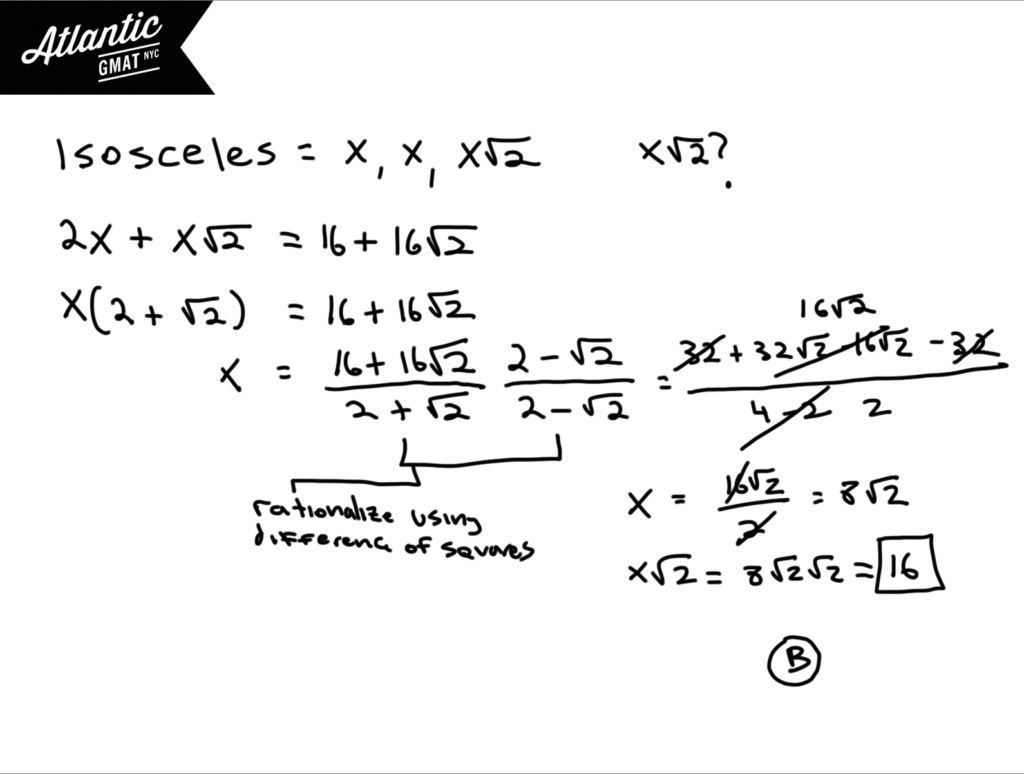You can also use the answer choices. I'd still do a bit of simplifying but then you can plug in the answers and see if the equation stays balanced (what is on the left equals what is on the right). Again, keep in mind that the answers are the hypotenuse, x√2, not x. So in order to plug them in for x we need to divide them by √2. Sounds more complicated than it is. Plugging in answers ends up being even easier than the algebra.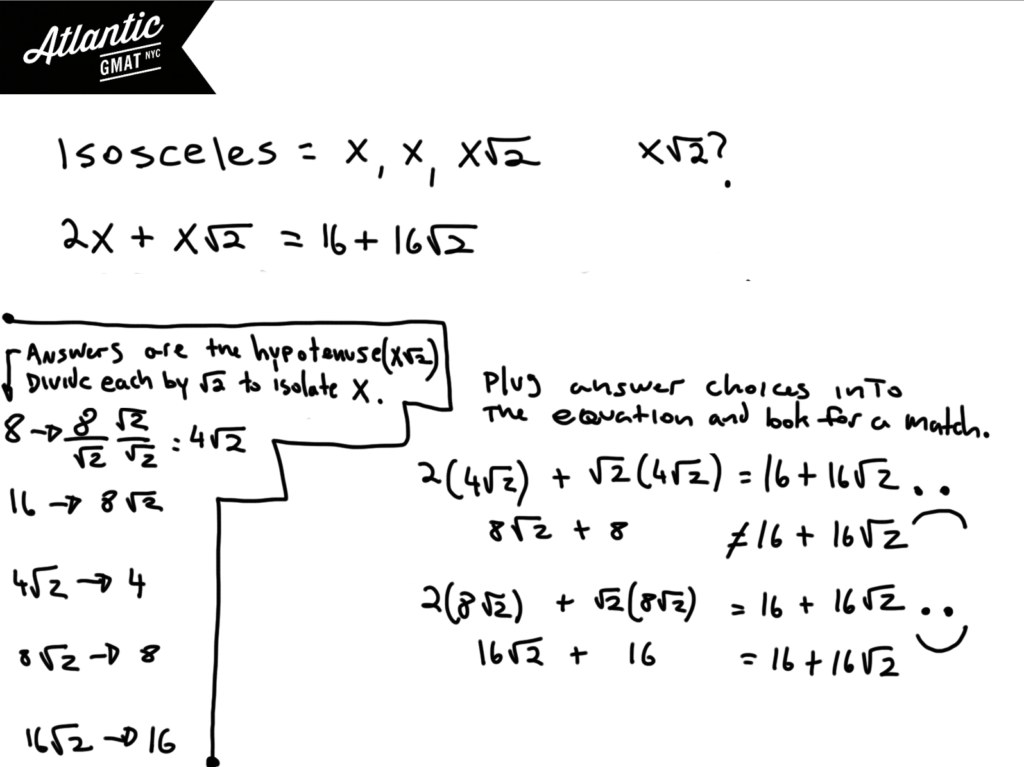Last but not least you can estimate. Estimation is a very helpful tool for getting through some GMAT questions. My advice is:

1. Keep your approximation/estimation in line with how far apart the answer choices are. In this case our approximations are within 10% and the closest answers are about 30% apart giving us some breathing room.
2. Use estimation/approximation as a useful tool deployed when appropriate not as a crutch for when you're in panic mode.

Here we just have to call √2 1.4. After that the arithmetic is basic. You can multiply everything by 10 to get rid of the decimals and then simplify. 16/17 is so close to 1 that we can just call it 1. 160/17 is very close to 10. With that we get 17. But we know that we're a tiny bit less than 17. The only answer that's remotely close is 16. 16√2 is almost 24. 8√2 is little less than 12. Those are way too far off to be considered. Comment with any questions or addition. Happy studies!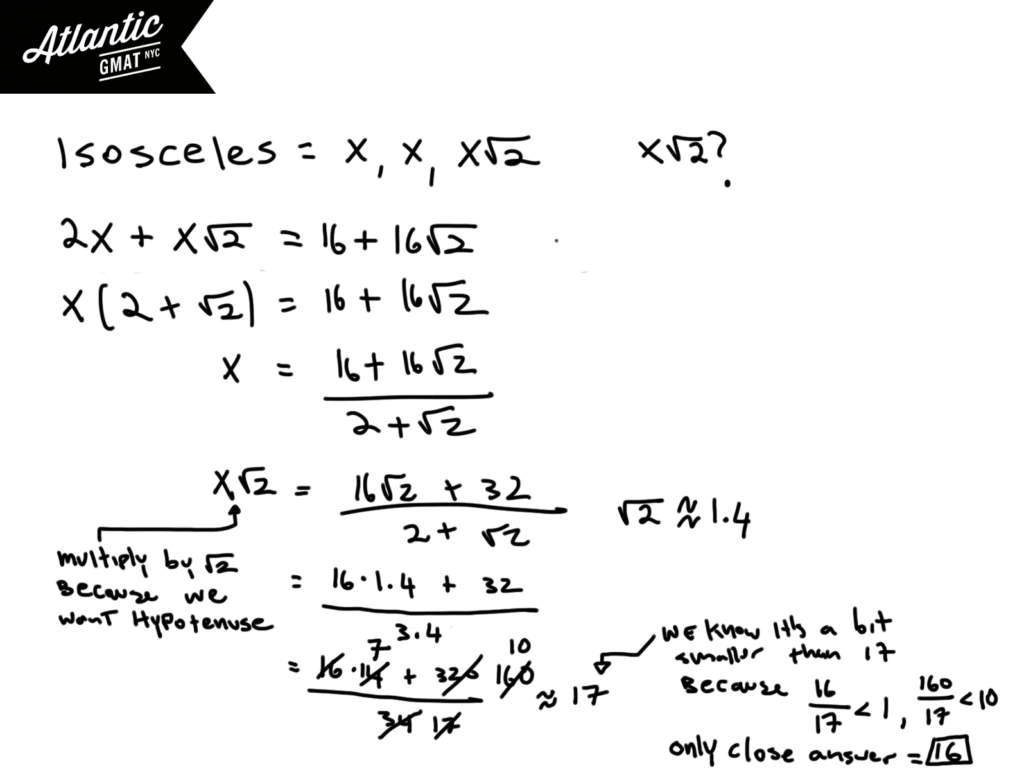A certain city with population of 132,000 is to be divided into 11 voting districts, and no district is to have a population that is more than 10 percent greater than the population of any other district. What is the minimum possible population that the least populated district could have?

A. 10,700
B. 10,800
C. 10,900
D. 11,000
E. 11,100

Read GMAT word problems carefully! Don't rush. Think as you read. Stop. Digest. Re-read pieces that have you scratching your head. A lot of this test be it the verbal or quant is about reading and critical thinking. Apply those skills to your quant work as you would to your verbal. For this question it's key to notice that you want to minimize one of the districts. Great - how do you do that? Maximize everything else! Are there any constraints? Yes! "No district is to have a population that is more than 10% greater than the population of any other district". Any other constraints? Nope. Alright, let's put pen to paper and translate the words to algebra (here's a video solution, a certain city with population of 132000). We have an equation with the districts on one side and 132,000 (total population) on the other: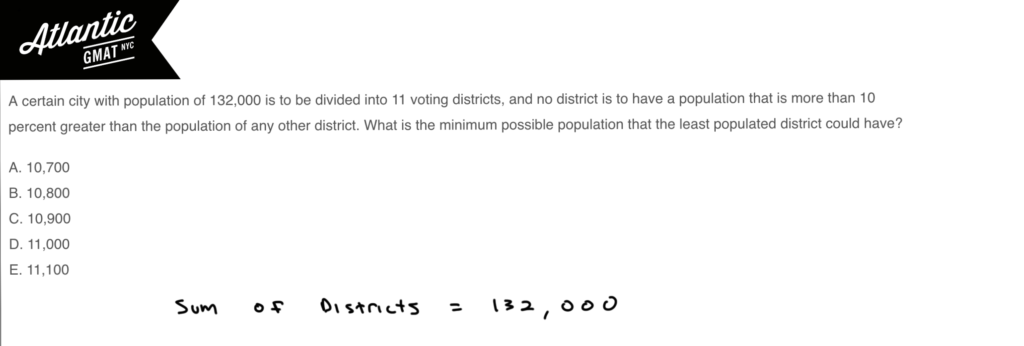Now let's apply the idea that we want to maximize 10 of these districts with the constraint that they can only be 10% greater than any other. Hmmm. How are you gonna do that? Let's create a variable for the smallest district (let's call it S). So how much greater can each of the other districts be? 1.1S (10% greater). You have have 10 of those. Now we can wrap up our equation and solve for S: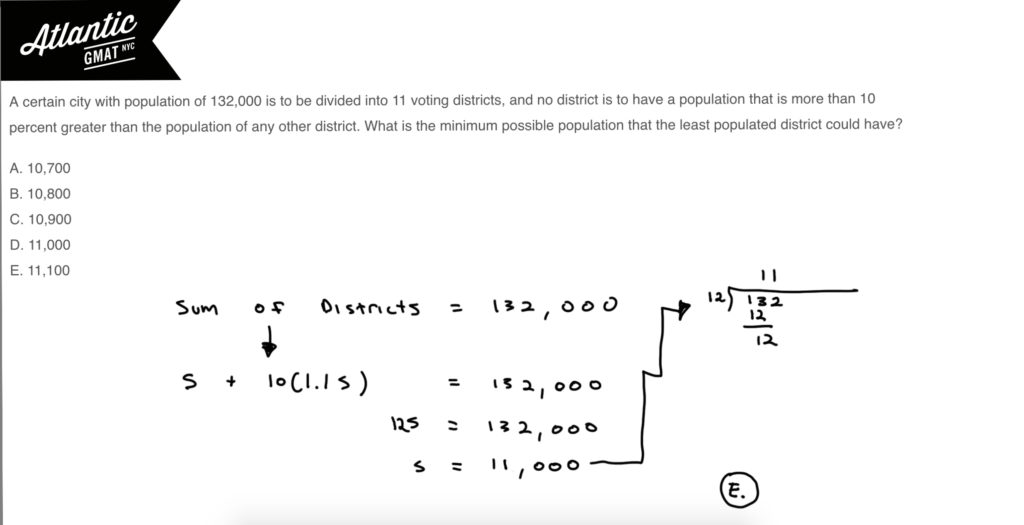Easy right? Now, you might say "yes it was easy but I wouldn't have known how to start". That's possible. Active reading, organized critical thinking, and translating to algebra takes consistent quality practice. Focus on these things through 200 GMAT questions over a period of a few weeks (recycling anything that you have trouble with several times using an error log) and you'll see progress. Again, here's a video solution: a certain city with population of 132000 Comment with any questions or additions!

For which of the following functions is f(a+b) = f(b) + f(a) for all positive numbers a and b?

A. f(x) =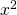B. f(x) = x+1
C. f(x) = √x
D. f(x) = 2/x
E. f(x) = -3x

Deja vu! Here's another function question from the GMAT prep. If you want to check out another solution for a functions question before tackling this one go here: For which of the following functions f(x)=f(1-x) and here's another extremely challenging GMAT function question that's a bit different and more on the puzzle side of things: For every positive even integer n the function h(n) is defined to be the product

Again, function questions generally look harder than they are. The first read is usually confusing. Just keep in mind that the premise is simple. For this one: find the answer choice for which plugging in a + b into the function is the same as plugging in a then plugging in b and summing the outcome. Let's just go through the answer choices. I would pick values for a and b. Let's go for a = 2, b = 3. Just a recap we want f(2 + 3) = f(2) + f(3). You can find a video explanation here: For which of the following functions is f(a+b) = f(b) + f(a) for all positive numbers a and b?

A. f(x) =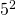vs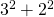25 vs 13

B. f(x) = x+1

5 + 1 vs (2+1) + (3+1)

6 vs 7

C. f(x) = √x

√5 vs. √2 + √3

√5 is less than 3. √2 is about 1.4, √4 is about 1.7 so their sum is more than 3.

D. f(x) = 2/x

2/5 vs 2/2 + 2/3

The right side is clearly bigger.

E. f(x) = -3x

-3(5) vs -3(2) + -3(3)

-15 = -15For which of the following functions f is f(x) = f(1-x) for all x?

A. f(x) = 1 - x

B. f(x) = 1 -C. f(x) =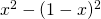D. f(x) =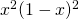E. f(x) = 1/(1-x)

My GMAT tutoring students often dislike these function questions. They see f(x) and the brain shuts down: pinwheel of doom (on mac) or BSOD (blue screen of death) on windows. I think it's tough to grasp exactly what you need to do from the first read. That's OK and an important thing to get used to. Go back and read it again. Think. Jot some things down. Challenge yourself. I think you'll find most of these function questions are rather basic. No complicated math. No tricks. Ok, so what does f(x) = f(1-x) mean? We just need to find the answer choice for which plugging in x yields the same value as plugging in 1 - x. Let's say x is 4. Then the function must yield the same value for 4 as for 1 - 4 (-3). Easy? It really is that easy. For this style of function question pick numbers. I'd probably avoid 1 (just my own superstition) but pretty anything is fair game. Let's say X is 3. Let's go through the answers and find the one for which plugging in 3 and 1 - 3 gives the same value. You can also find a video explanation here: For which of the following functions f is f(x) = f(1-x) for all x?

A. f(x) = 1 - x

x = 3

1-3 = -2

x = -2

1-(-2) = 3

B. f(x) = 1 -x = 3

1-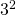= -8

x = -2

1 -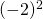= -3

C. f(x) =x = 3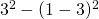= 5

x = -2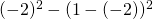= -5

D. f(x) =x = 3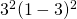= 36

x = -2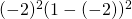= 36

E. f(x) = 1/(1-x)

x = 3

1/(1-3) = -1/2

x = -2

1/(1-(-2)) = 1/3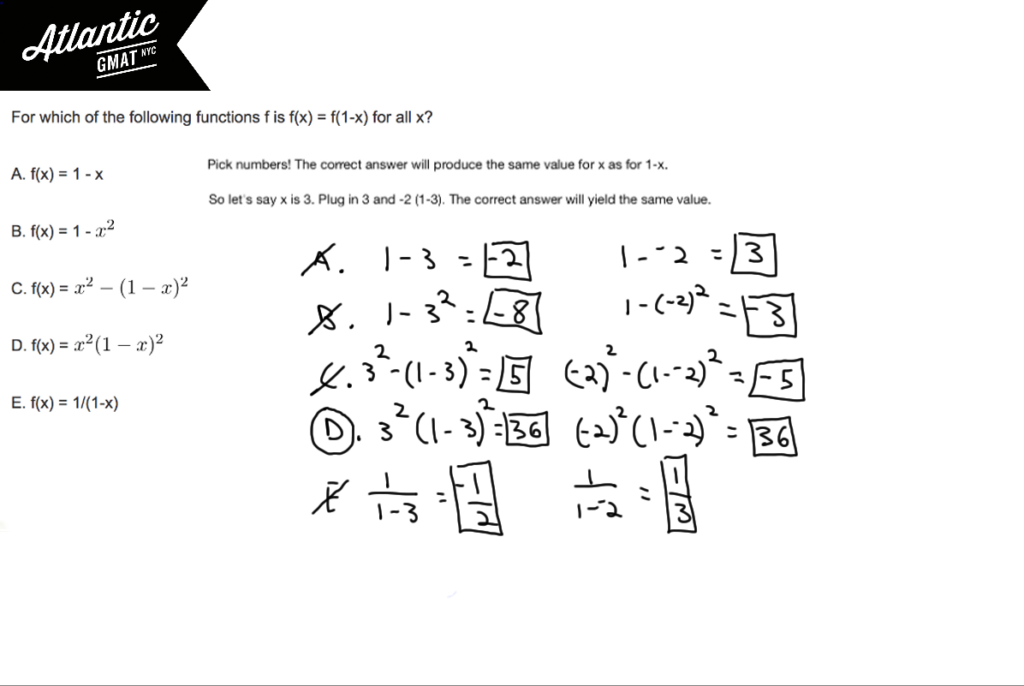This function question is relatively common. Usually plugging in numbers makes it easy to solve. Here are two more GMAT Function questions. The first one is very similar to this one while the second adds some number properties to the mix and is on of the most challenging questions in the GMAT universe:

For which of the following functions is f(a+b) = f(b) + f(a) for all positive numbers a and b?

For every positive even integer n the function h(n) is defined to be the product

Remember: Don't rush the follow through. Do your arithmetic carefully. That doesn't mean quadruple check everything. Just go at the pace that makes sense for you so that you can produce accurate calculations consistently. Comment away with any questions or concerns!

## GMAT ARTICLES

Atlantic GMAT

405 East 51st St.

NY, NY 10022

(347) 669-3545

info@AtlanticGMAT.com